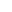Though we utilize a basic Western Wedding Style format similar to what you would see in a movie, they are flexible in so many ways – we can make them simple, extravagant, exotic or somewhere in between, the choice is totally yours.

E-mail address not matched with above.

Invalid Telephone Number.

Invalid Input

(Please uncheck what you don't need to be included)

Invalid Input

Invalid Input

Invalid Input

Invalid Input

Invalid Input

Invalid Input

Invalid Input

Invalid Input

Invalid Input

Invalid Input

Invalid Input

Invalid Input

Invalid Input

Invalid Input

Invalid Input

Invalid Input

Invalid Input

Invalid Input

Invalid Input

Invalid Input

Invalid Input

Invalid Input

Invalid Input

Invalid Input

Invalid Input

Invalid Input

Invalid Input

Invalid Input

Invalid Input

Invalid Input

Invalid Input

Invalid Input

Invalid Input

Invalid Input

Invalid Input

Invalid Input

Invalid Input

Invalid Input

Invalid Input

Invalid Input

Invalid Input

Invalid Input

Invalid Input

Invalid Input

Invalid Input

Invalid Input

Invalid Input

Invalid Input

Invalid Input

Invalid Input

Invalid Input

Invalid Input

Invalid Input

Invalid Input

Invalid Input

Invalid Input

Invalid Input

Invalid Input

Invalid Input

Invalid Input

Invalid Input

Invalid Input

Invalid Input

Invalid Input

Invalid Input

Invalid Input

Invalid Input

Invalid Input

Invalid Input

Invalid Input

Invalid Input

Invalid Input

Invalid Input

Invalid Input

Invalid Input

Invalid Input

Invalid Input

Invalid Input

Invalid Input

Invalid Input

Invalid Input

Invalid Input

Invalid Input

Invalid Input

Invalid Input

Invalid Input

Invalid Input

Invalid Input

Invalid Input

Invalid Input

Invalid Input

Invalid Input

Invalid Input

Invalid Input

Invalid Input

Invalid Input

Invalid Input

Invalid Input

Invalid Input

Invalid Input

Please let us know how and when to contact you.

Please select a date when we should contact you.

Invalid Input

User Rating: 5 / 5